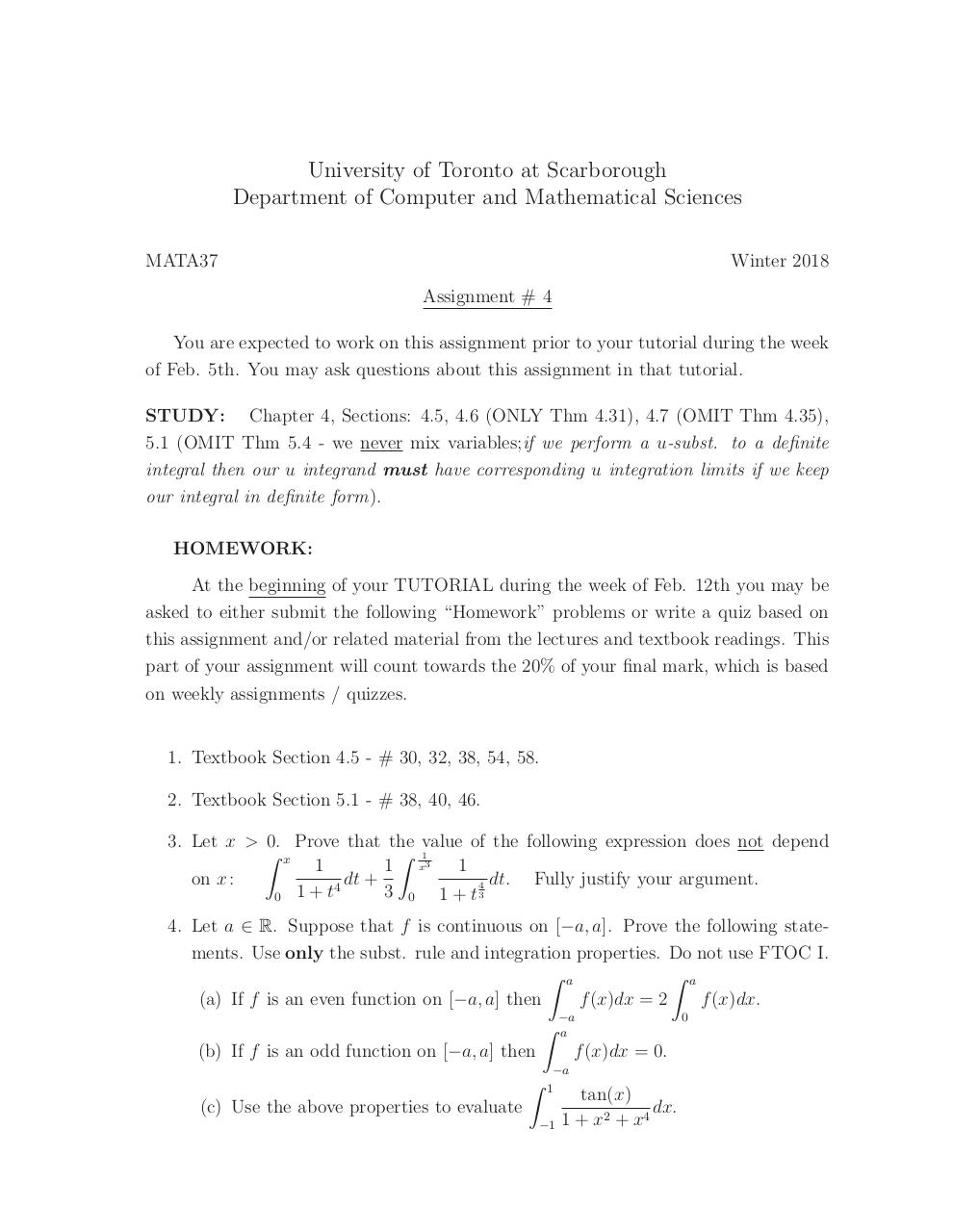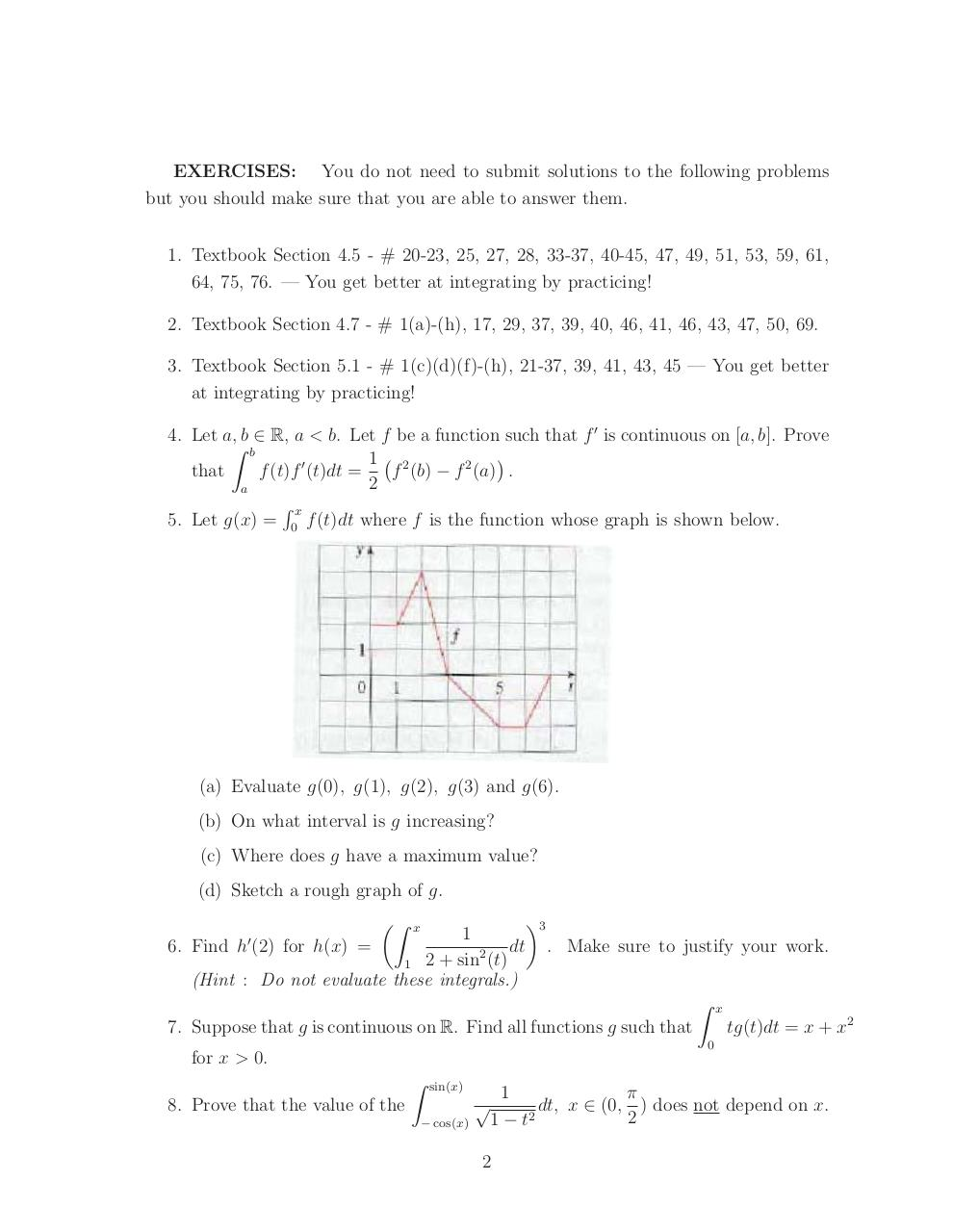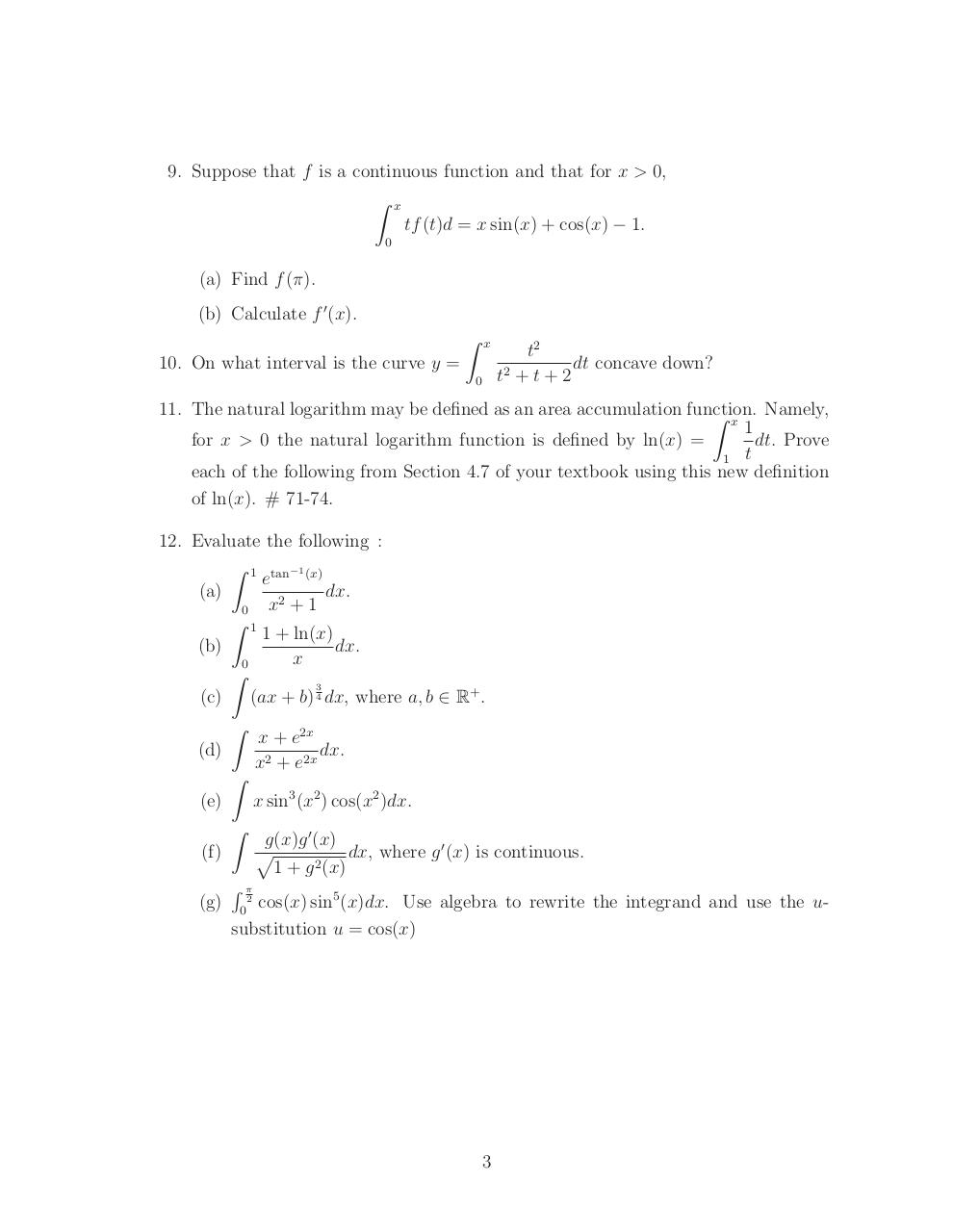# A4 W18 w picture .pdf

### File information

Original filename: A4 W18 w picture.pdf
Title: A4 W18 w pic.pdf
Author: Kathleen

This PDF 1.5 document has been generated by TeX / Acrobat Distiller 11.0 (Windows), and has been sent on pdf-archive.com on 09/02/2018 at 19:33, from IP address 142.151.x.x. The current document download page has been viewed 419 times.
File size: 58 KB (3 pages).
Privacy: public file

A4 W18 w picture.pdf (PDF, 58 KB)

### Document preview

University of Toronto at Scarborough
Department of Computer and Mathematical Sciences
MATA37

Winter 2018
Assignment # 4

You are expected to work on this assignment prior to your tutorial during the week
STUDY: Chapter 4, Sections: 4.5, 4.6 (ONLY Thm 4.31), 4.7 (OMIT Thm 4.35),
5.1 (OMIT Thm 5.4 - we never mix variables;if we perform a u-subst. to a deﬁnite
integral then our u integrand must have corresponding u integration limits if we keep
our integral in deﬁnite form).
HOMEWORK:
At the beginning of your TUTORIAL during the week of Feb. 12th you may be
asked to either submit the following “Homework” problems or write a quiz based on
this assignment and/or related material from the lectures and textbook readings. This
part of your assignment will count towards the 20% of your ﬁnal mark, which is based
on weekly assignments / quizzes.

1. Textbook Section 4.5 - # 30, 32, 38, 54, 58.
2. Textbook Section 5.1 - # 38, 40, 46.
3. Let x &gt; 0. Prove that the value of the following expression does not depend
 x
 1
1
1
1 x3
dt +
on x :
4
3 0 1 + t 43
0 1+t
4. Let a ∈ R. Suppose that f is continuous on [−a, a]. Prove the following statements. Use only the subst. rule and integration properties. Do not use FTOC I.
 a
 a
(a) If f is an even function on [−a, a] then
f (x)dx = 2
f (x)dx.
−a
0
 a
(b) If f is an odd function on [−a, a] then
f (x)dx = 0.


−a
1

(c) Use the above properties to evaluate
−1

tan(x)
dx.
1 + x2 + x4

EXERCISES: You do not need to submit solutions to the following problems
but you should make sure that you are able to answer them.
1. Textbook Section 4.5 - # 20-23, 25, 27, 28, 33-37, 40-45, 47, 49, 51, 53, 59, 61,
64, 75, 76. — You get better at integrating by practicing!
2. Textbook Section 4.7 - # 1(a)-(h), 17, 29, 37, 39, 40, 46, 41, 46, 43, 47, 50, 69.
3. Textbook Section 5.1 - # 1(c)(d)(f)-(h), 21-37, 39, 41, 43, 45 — You get better
at integrating by practicing!
4. Let a, b ∈ R, a &lt; b. Let f be a function such that f  is continuous on [a, b]. Prove
 b

1 2
f (b) − f 2 (a) .
f (t)f  (t)dt =
that
2
a
x
5. Let g(x) = 0 f (t)dt where f is the function whose graph is shown below.

(a) Evaluate g(0), g(1), g(2), g(3) and g(6).
(b) On what interval is g increasing?
(c) Where does g have a maximum value?
(d) Sketch a rough graph of g.


x

1
6. Find h (2) for h(x) =
dt
2
1 2 + sin (t)
(Hint : Do not evaluate these integrals.)


3
. Make sure to justify your work.


7. Suppose that g is continuous on R. Find all functions g such that
for x &gt; 0.


sin(x)

8. Prove that the value of the
− cos(x)

x

tg(t)dt = x + x2

0

1
π
dt, x ∈ (0, ) does not depend on x.
2
1 − t2

2

9. Suppose that f is a continuous function and that for x &gt; 0,


x
0

tf (t)d = x sin(x) + cos(x) − 1.

(a) Find f (π).
(b) Calculate f  (x).


x

10. On what interval is the curve y =
0

t2
dt concave down?
t2 + t + 2

11. The natural logarithm may be deﬁned as an area accumulation function.
 x Namely,
1
for x &gt; 0 the natural logarithm function is deﬁned by ln(x) =
dt. Prove
1 t
each of the following from Section 4.7 of your textbook using this new deﬁnition
of ln(x). # 71-74.
12. Evaluate the following :
 1 tan−1 (x)
e
(a)
dx.
x2 + 1
0
 1
1 + ln(x)
dx.
(b)
x
0

3
(c) (ax + b) 4 dx, where a, b ∈ R+ .

x + e2x
(d)
dx.
x2 + e2x

(e)
x sin3 (x2 ) cos(x2 )dx.

g(x)g  (x)

dx, where g  (x) is continuous.
(f)
2
1 + g (x)

(g) 02 cos(x) sin5 (x)dx. Use algebra to rewrite the integrand and use the usubstitution u = cos(x)

3#### HTML Code

Copy the following HTML code to share your document on a Website or Blog

#### QR Code### Related keywords